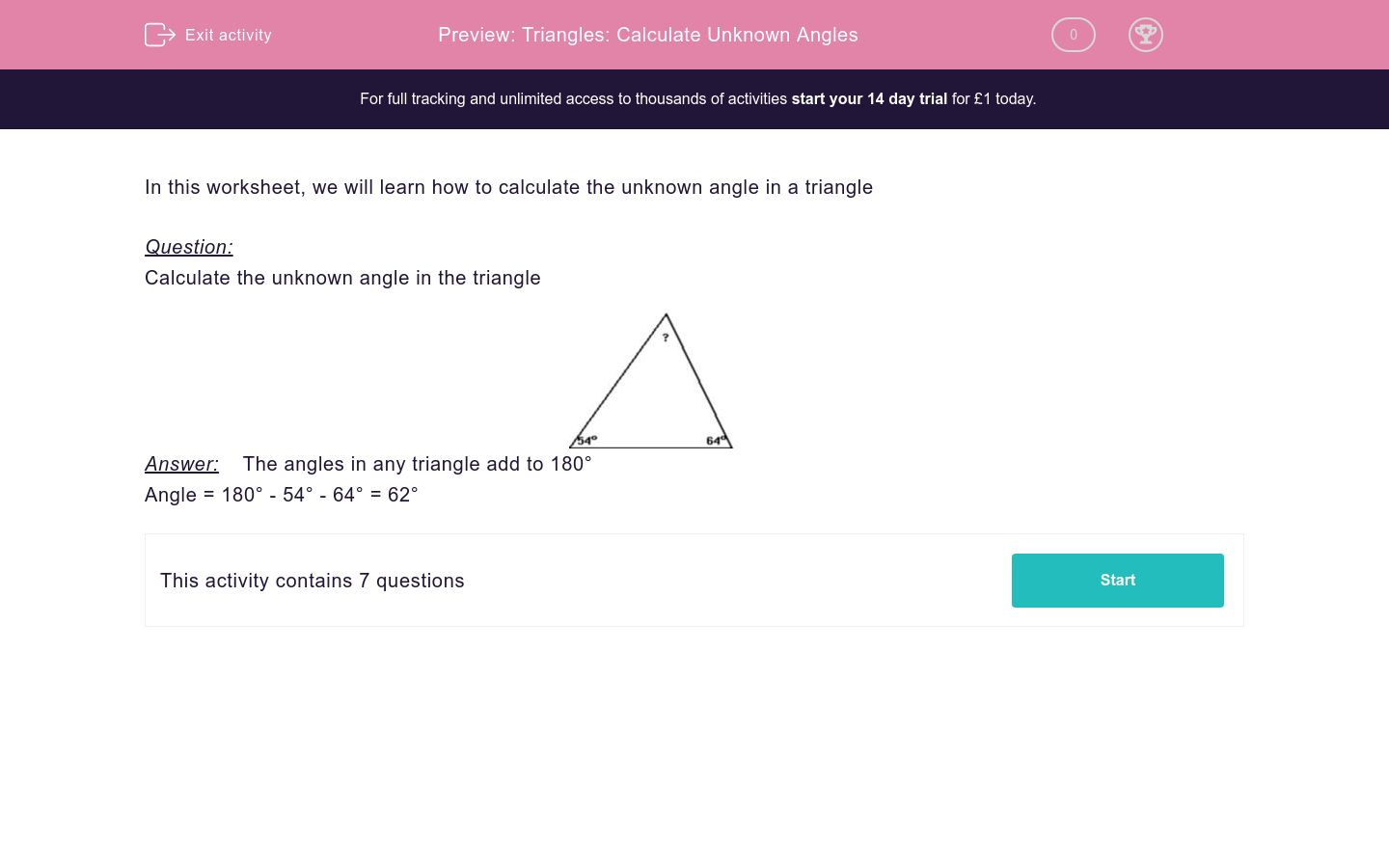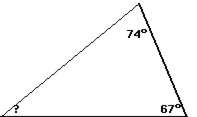# Triangles: Calculate Unknown Angles

In this worksheet, students use their knowledge of the properties of a triangle to evaluate unknown angles.Key stage:  KS 2

Curriculum topic:   Maths and Numerical Reasoning

Curriculum subtopic:   2D Shapes: Triangles, Quadrilaterals and Polygons

Difficulty level:### QUESTION 1 of 10

In this worksheet, we will learn how to calculate the unknown angle in a triangle

Question:

Calculate the unknown angle in the triangleAngle = 180° - 54° - 64° = 62°

Calculate the unknown angleCalculate the unknown angleCalculate the unknown angleCalculate the unknown angleCalculate the unknown angleCalculate the unknown angleCalculate the unknown angle• Question 1

Calculate the unknown angle37
EDDIE SAYS
180 - 77 - 66 = 37
• Question 2

Calculate the unknown angle32
EDDIE SAYS
180 - 69 - 79 = 32
• Question 3

Calculate the unknown angle41
EDDIE SAYS
180 - 87 - 52 = 41
• Question 4

Calculate the unknown angle39
EDDIE SAYS
180 - 74 - 67 = 39
• Question 5

Calculate the unknown angle74
EDDIE SAYS
180 - 22 - 84 = 74
• Question 6

Calculate the unknown angle64
EDDIE SAYS
180 - 90 - 26 = 64
• Question 7

Calculate the unknown angle25
EDDIE SAYS
180 - 86 - 69 = 25
---- OR ----

Sign up for a £1 trial so you can track and measure your child's progress on this activity.

### What is EdPlace?

We're your National Curriculum aligned online education content provider helping each child succeed in English, maths and science from year 1 to GCSE. With an EdPlace account you’ll be able to track and measure progress, helping each child achieve their best. We build confidence and attainment by personalising each child’s learning at a level that suits them.

Get started